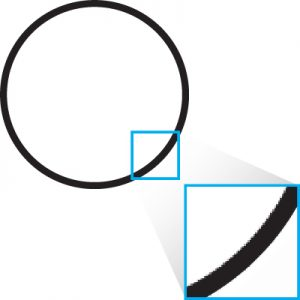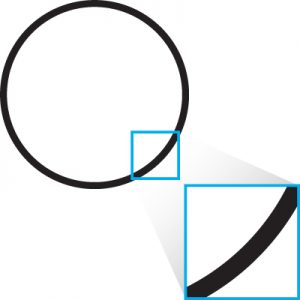# The Differences Between Vector and Raster Images

### Raster ImagesWhat is a Raster file? Raster images are created from pixels, which are small colored squares that make up the image. This means that as you increase the size of the image, it will eventually become blocky (pixelated), or appear to be blurred.

Common Raster File Formats: .jpg, .jpeg, .gif, .png

Pros of Raster Images: Even though raster images become visibly pixelated and/or blurry when increasing the image size, there are still a few positive aspects of these image files. As stated before, raster images are created by pixels and each individual pixel’s color can be modified. This provides a level of precision editing that is above Vector images.

Each raster image contains a “dpi”, or dots per inch count, which is the number of pixels in a 1” x 1” square of the image. The higher the dpi, the larger the image can be increased without becoming blocky. A higher dpi count also allows for a more detailed image.

Cons of Raster Images: No matter what the dpi count of the image is, every raster image will become pixelated at some point when increasing the image size. These image files also tend to be large. For example, consider the dpi count of the Raster image is 400 for a 1” x 1” square. If your image is 20” x 28”, there would be 224,000 pieces of information your computer would need to store.

### Vector ImagesWhat is a Vector file? Vector images are built from mathematically precise points. As you increase or decrease the size of the image, the mathematical equation is changed automatically to create a consistent shape. This creates an image that can be infinitely scaled without losing any image quality.

Common Vector File Formats: .ai, .eps, .pdf, .cdr

Pros of Vector Images: Vector images are infinitely scalable. This means that the quality of the image will remain consistent no matter how much the image size is increased.

Since Vector images use mathematical equations to create images, there is no need for pixels. Instead, this equation uses points to create the image, as opposed to individual pixels. This allows for a smaller file size.

Cons of Vector Images: Since Vector images use equations to fill in the missing information in an image, this equation is unable to fill in complex color palettes as well as raster images can.